# Overcome common challenges in GM (1,1) modelling using MS Excel

Grey theory deals with a system consisting of inadequate or improper information. It includes systems analysis, data processing, modelling, prediction, decision-making, and control. There are different grey models in use in many applications. Grey system theory was introduced by Julong (1989). GM (1,1) modelling is a popular grey forecasting method because of its computational efficiency. There are many challenges in GM (1,1) modelling, but they are solvable using MS Excel. This article is a detailed guide on how to overcome these challenges.

## Forms and uses of GM (1,1) modelling

There are four basic forms of model GM (1,1):

1. Even Model GM(1,1) (EGM).
2. Original Difference Model GM(1,1) (ODGM).
3. Even Difference Model GM(1,1) (EDGM).
4. Discrete Model GM(1,1) (DGM).

GM (1,1) modelling is very useful in forecasting the future outputs of the system as it has a high level of accuracy. One of the major advantages of GM (1,1) model is that it requires little data. Moreover, it is very popular with short-term forecasting activities. Prediction accuracy of GM (1,1) model is estimated by Mean Absolute Percentage Error (MAPE). Low values of MAPE means forecasted value from the grey model is very close to actual values. Generally, below 10 % MAPE confirms that the GM (1,1) provides a good prediction accuracy (Wang, Lin, Hsu, Le, & Lin, 2016). It also plays a major role in estimating and predicting efficiency values and benchmarking analysis.

## Challenges in GM (1,1) modelling

Following are the challenges with GM (1,1) modelling:

1. One of the major challenges in grey forecasting is that it involves solving a complex equation. This becomes more tiresome when there is a large number of entries and results.
2. It takes a lot of effort and time to calculate the prediction of outputs accurately.
3. After collecting the data, the first step in GM (1,1) model involves a transformation of an original sequence into a new sequence using a first-order accumulated generation operator.
4. In the steps for solving GM (1,1) model equations, one shortcoming is that it has some prerequisite condition that it follows a monotonic exponential prediction approach.
5. Although it is considered a good forecasting model it ignores new information and is not able to reflect the characteristics of the current situation accurately (Zhou & Zhang, 2016).
6. Expertise in different software like MATLAB, UCINET, and languages like R is required.

Below are a few steps to address the above-mentioned challenges.

## Solving complex equations

Manually conducting GM (1, 1) is time-consuming, complex, and fraught with errors. Therefore follow each step below in order to overcome the complexity of equations. Let’s consider an example where there are 3 variables for the year 2012-2015. This will show that GM (1,1) model will help in determining prediction values for the future time period from 2016-2019.

### Step 1

It involves the collection of the data. The original time series dataset is presented as X(0).

`X(0) =  (X(0) (0), X(0) (1), X(0) (2), X(0) (3)..........,X(0) (n))`Figure 1: Step 1 for solving a complex equation in GM (1,1) modelling

### Step 2

There will be a specific calculation for each input and output variable. This demonstration shows only the calculation for Input (I).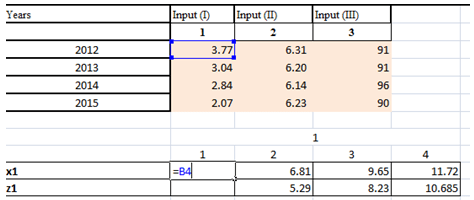Figure 1: Step 2 for solving a complex equation in GM (1,1) modelling

Derive the accumulated time series data X(1)

X(1) =  (X(1) (1), X(1) (2), …………,X(1) (n))

Where, X(1) (1) = X(0) (1) if k = 1

X(1)(k) = (i)   if k = 2, . . . , n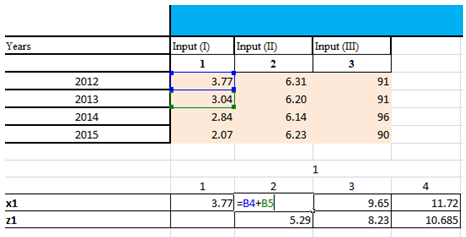Figure 3: Calculating time series data

### Step 3

Then, the mean sequence Z(1) of X(1) can be derived as

```Z(1) = (Z(1)(2) , Z(1) (3) ,...., Z(1) (n))

where Z(1)(k) is the mean value of two adjacent data

Z(1)(k) =  X (1)(k)+ X (1)(k−1)) ,  k=2,3,.....n```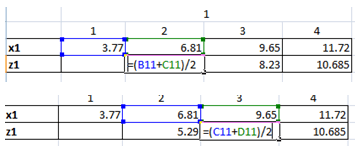Figure 4: Step 3 (calculating mean sequence) of solving complex equation in GM (1,1) modelling

### Step 4

Convert the linear equations into the form of a matrix B.Figure 4: Converting to linear equations

### Step 5

Then BT  (transpose of matrix B) will be estimated using the following formula;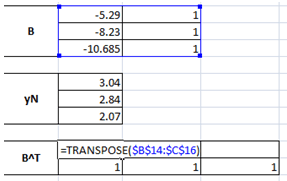Figure 5: Transposing of matrix B

### Step 6

From X(1) ,  a GM(1,1) model which corresponds to the first order differential equation X(1) (k) can be constructed as follows;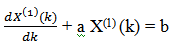where parameters a and b are called the developing coefficient and grey input, respectively.

### Step 7

Then  [a, b]T = (BT B)−1BTY is calculated using the following formulas;Figure 6: Calculating the data matrix

The vector Y is called the data series, YN   = ( 3.04, 2.84 , 2.07 )T.

Vector B is called the data matrix, and [a, b]T is called the parameter series.

### Step 8

Now, substitute the different value of k into the equation;X(1) (1) = 3.77  when k= 0 and X(1) (2) = 6.893 when k = 1Figure 7: Substituting the K value

### Step 9

Next step is to derive the predicted value of the original series, according to the accumulated generating operation.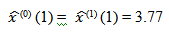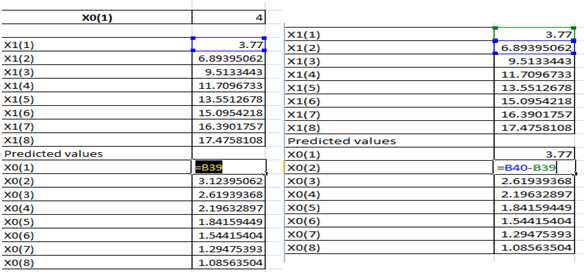Figure 8: Predicting the value of the original series

As with above process, we have the results for all variables from period 2016-2019.

x(0)(5) =X (1) (5) – X (1) (4) –  Result forecast of 2016

x(0)(6) =X (1) (6) – X (1) (5) –   Result forecast of 2017

x(0)(7) =X (1) (7) – X (1) (6) –   Result forecast of 2018

x(0)(8) =X (1) (8) – X (1) (7) –  Result forecast of 2019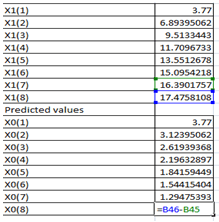Figure 9: Forecasted results

x(0)(7) =X (1) (7) – X (1) (6) –   Result forecast of 2018

x(0)(8) =X (1) (8) – X (1) (7) –  Result forecast of 2019Figure 10: Forecasted results

## Data fragmentation and curation

After collecting the data it is very important to curate and transform the raw data. Otherwise, the manual implications of the equations of the data will not generate appropriate results. In order to do so, follow the steps and the equations mentioned in Steps 2 & 3. Initially, derive the accumulated time series data and then the mean values.

## Information exchange and presentation

In some software, the model ignores new information and is not able to reflect the characteristics of the current situation accurately. This is a major challenge which one can avoid by following the steps above (step 1-9).

## Software related challenge

As mentioned in the earlier sections there is various software that allows conduction of grey forecasting. Some of the most prominent are, MATLAB, and R-Studio (R language). The challenge is that a person with no idea of languages and high-end software will never be able to conduct grey forecasting. Therefore, even if conducting grey forecasting in MS Excel is tiresome and time-consuming, but is easy to apply and effective results.

## Calculating future years

The first mistake one may commit is forecasting the same years of analysis. This is because the equation, in this case, is a bit tricky.

For example, in a dataset of observations for the period 2010 to 2015, if the equations are incorrect then the model may end up forecasting for 2010-2015 instead of 2016-2020.

However, this is possible, if the steps 7, 8, & 9 are followed. Similarly, using sample data from period 2012-2015, one can predict the values for the future period 2016-2019.

Therefore, GM (1,1) model is a flexible approach to predict the future values. Most of the studies have shown that results from GM (1,1) model will be more accurate and it can also easily handle a time series data with a small sample size. The next article discusses Super-SBM efficiency model in detail helps measure efficiency. It provides efficiency score which is units invariant and a monotone function of input slacks and output slacks, thus giving it the name “super slack based measure”.

#### References

• Julong, D. (1989). Introduction to Grey System Theory. The Journal of Grey System1, 1, 1–24. https://doi.org/0957-3720.
• Wang, C. N., Lin, H. S., Hsu, H. P., Le, V. T., & Lin, T. F. (2016). Applying data envelopment analysis and grey model for the productivity evaluation of Vietnamese agroforestry industry. Sustainability (Switzerland), 8(11). https://doi.org/10.3390/su8111139.
• Zhou, W., & Zhang, D. (2016). An Improved Metabolism Grey Model for Predicting Small Samples with a Singular Datum and Its Application to Sulfur Dioxide Emissions in China. Discrete Dynamics in Nature and Society, 2016. https://doi.org/10.1155/2016/1045057.

### Avishek Majumder

Research Analyst at Project Guru
Avishek is a Master in Biotechnology and has previously worked with Lifecell International Private Limited. Apart from data analysis and biological research, he loves photography and reading. He loves to play football and basketball in his spare time with an avid interest in adventure and nature. He was also a member of the Scouts in his school and has attended Military training.

#### Latest posts by Avishek Majumder (see all)We are looking for candidates who have completed their master's degree or Ph.D. Click here to know more about our vacancies.